Function Repository Resource:

# PermutationMajorIndex

Compute the major index of a permutation

Contributed by: Wolfram Staff (original content by Sriram V. Pemmaraju and Steven S. Skiena)
 ResourceFunction["PermutationMajorIndex"][p] gives the major index of the permutation p.

## Details and Options

The major index of a permutation p={p1,p2,} is the sum of the positions of the descents of the permutation. A descent occurs at position j when pj>pj+1.

## Examples

### Basic Examples (2)

Since descents of this permutation are at positions 2 and 4, the major index is 2+4=6:

 In:=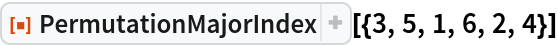Out=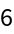There are six permutations of length 4 with major index 3:

 In:=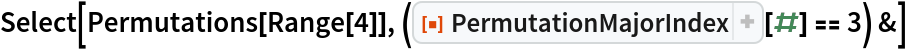Out=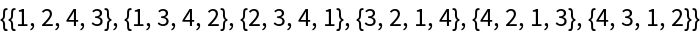In:=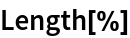Out=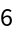There is an equal number of permutations of length 4 with three inversions:

 In:=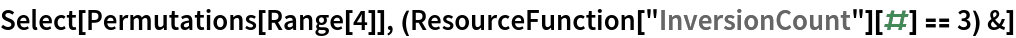Out=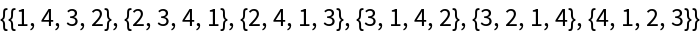In:=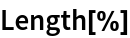Out=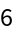### Properties and Relations (1)

The number of permutations of length n with major index k and inversion count i is the same as the number of permutations of length n with major index i and inversion count k:

 In:=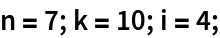In:=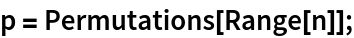In:=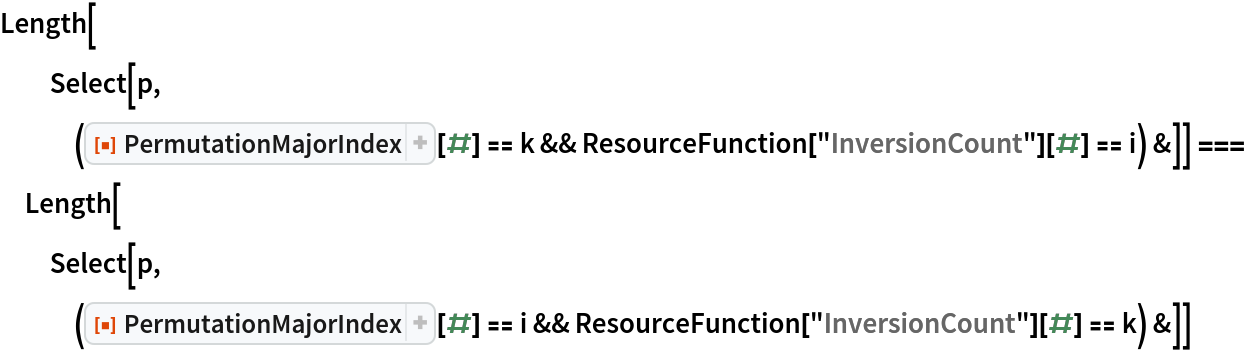Out=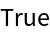### Neat Examples (1)

The number of permutations of length 4 with given major index and inversion count:

 In:=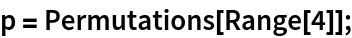In:=Out=## Version History

• 1.0.0 – 06 July 2020